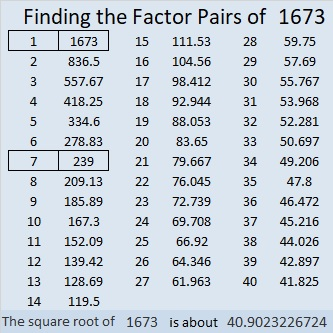# 1673 and Level 1

Contents

### Today’s Puzzle:

Write the numbers from 1 to 10 in both the first column and the top row so that those numbers and the given clues become a not-in-the-usual-order multiplication table.### Factors of 1673:

• 1673 is a composite number.
• Prime factorization: 1673 = 7 × 239.
• 1673 has no exponents greater than 1 in its prime factorization, so √1673 cannot be simplified.
• The exponents in the prime factorization are 1 and 1. Adding one to each exponent and multiplying we get (1 + 1)(1 + 1) = 2 × 2 = 4. Therefore 1673 has exactly 4 factors.
• The factors of 1673 are outlined with their factor pair partners in the graphic below.### More About the Number 1673:

The factors of 1673 are 1, 7, 239, and 1673. OEIS.org tells us something cool about the sum of the squares of those factors:
1² + 7² + 239² + 1673² = 1690².

This site uses Akismet to reduce spam. Learn how your comment data is processed.